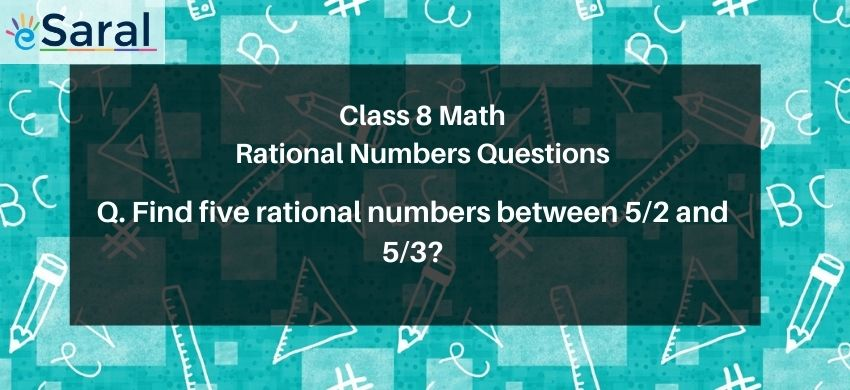Most Affordable JEE | NEET | 8,9,10 Preparation by Kota's Top IITian Doctor Faculties

# Find two rational numbers between 2/3 and 5/3# Question. Two rational numbers between $\frac{2}{3}$ and $\frac{5}{3}$ are:

1. $\frac{1}{6}$ and $\frac{2}{6}$
2. $\frac{1}{2}$ and $\frac{2}{1}$
3. $\frac{5}{6}$ and $\frac{7}{6}$
4. $\frac{2}{3}$ and $\frac{4}{3}$

Solution:

Correct option is 3
$\frac{5}{6}$ and $\frac{7}{6}$
Changing the denominators of both numbers to 6, we get
$\frac{2}{3}=\frac{4}{6} \quad \& \quad \frac{5}{3}=\frac{10}{6}$
Numbers between the given rational numbers from the options are
$\frac{5}{6} \quad \& \quad \frac{7}{6}$
So, correct answer is option 3.